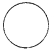• Circle
• Triangle
• Square
• Any shape

• One
• Two
• Three
• Four

• Tube
• A tent
• A ball
• A box

• Circle
• Square
• Rectangle
• Triangle

• Circle
• Square
• Rectangle
• Triangle

• cylinder
• square
• circle
• triangle

• sphere
• cylinder
• cone
• triangle

• cone
• sphere
• cuboid
• cylinder

• triangle
• cone
• cylinder
• sphere
• #### 10.  The name of the shape is• cone
• circle
• cylinder
• cube
Report Question
Practice More On Visualising Solid Shapes
access_time